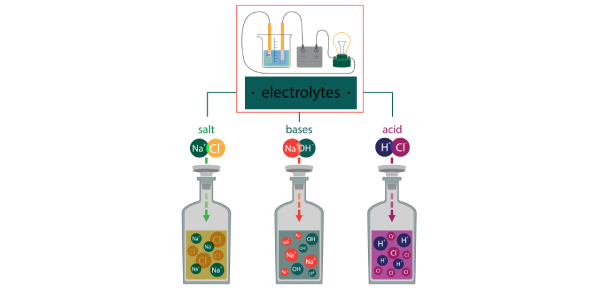# Acid Base And Salt Quiz Questions And Answers

20 Questions | Total Attempts: 10940SettingsCreate your own QuizHave you studied acids, bases, and salts in Chemistry? Then why not take this acid, base, and salt quiz we have specially prepared for you. Our quiz can be perfect if you want a way to test out just how much you know about them. For you to pass this quiz, you will need to have some knowledge when it comes to formulas of different acid salts and base and their properties. Give it a shot, and feel free to take the quiz as much as you need and share it with others.

• 1.
Which of the following can form two acid salt?
• A.

HCl

• B.

HNO3

• C.

H2SO4

• D.

H3PO4

• 2.
Which of the following acid will dissociate completely in an aqueous solution?
• A.

Hydrochloric acid

• B.

Carbonic acid

• C.

Acetic acid

• D.

Formic acid

• 3.
Which of the following acid is a dibasic acid?
• A.

HCOOH

• B.

H2SO4

• C.

HNO3

• D.

HNO2

• 4.
Which of the following is a diacidic base?
• A.

Sodium hydroxide

• B.

Potassium hydroxide

• C.

Ammoniumhydroxide

• D.

Calciumhydroxide

• 5.
Which of the following is an acid salt?
• A.

K2SO4

• B.

(NH4)2SO4

• C.

KH2PO4

• D.

K3PO4

• 6.
Which of the following statement is wrong?
• A.

A weak acid dissociates incompletely.

• B.

The solution of a salt of a weak acid and strong base is alkaline.

• C.

The solution of a salt of a weak acid and a strong base is neutral.

• D.

A monobasic acid cannot form an acid salt.

• 7.
An efflorescent salt
• A.

Is anhydrous salt that absorbs moisture from the air

• B.

Is a hydrated salt that loses its water to the atmosphere

• C.

Turns blue litmus red

• D.

Turns red litmus blue

• 8.
Which of the following oxide is not an alkali?
• A.

Ammonium hydroxide

• B.

Calcium hydroxide

• C.

Copper hydroxide

• D.

Sodium hydroxide

• 9.
The more acidic a solution, the higher is its pH.
• A.

True

• B.

False

• 10.
In a solution with pH-13, ammonium salt is added and gently heated.
• A.

It will liberate a gas that is acidic in nature and turns moist litmus paper red

• B.

It will liberate basic gas, which gives dense white fume when a rod dipped in HCl is brought near it.

• C.

Precipitate forms, which is insoluble in all mineral acid

• D.

No reaction takes place

• 11.
Two sulphate which are insoluble (choose the correct pair)
• A.

• B.

Ammonium sulphate/sodium sulphate

• C.

Barium sulphate/lead (II) sulphate

• D.

Sodium sulphate/ potassium sulphate

• 12.
A given white crystalline salt was tested as follows: a. It dissolves in water, and the resulting solution of the salt turns blue litmus red b. The addition of barium chloride solution into this solution gives a white precipitate c. A flame test on the salt gave a persistent golden-yellow colorisation. Identify the salt
• A.

Potassium sulphate

• B.

Ammonium sulphate

• C.

Sodium sulphate

• D.

Sodium chloride

• 13.
"Conc. H2SO4, when reacted with sodium chloride, liberates HCl gas as the product " Which property of conc. sulphuric acid is depicted by the mentioned reaction
• A.

Oxidizing nature

• B.

Acidic nature

• C.

Non-volatile nature

• D.

Both acidic and oxidizing nature

• 14.
Choose the correct option from the following.
• A.

Universal indicator is a class of compound that shows distinctly different colors in acidic and alkaline solution.

• B.

Acid-Base indicators show different colors at different pH values.

• C.

A universal indicator is class of compounds that shows different colors at different pH values.

• D.

Methyl orange is a universal indicator.

• 15.
Sodium carbonate, when treated with a solution, liberates a gas that turns lime-water milky and has no effect on acidified potassium dichromate solution. Choose the correct conclusion from the above observation.
• A.

The pH of the solution less than 7

• B.

Gas liberated is carbon dioxide

• C.

Both the above observation is correct

• D.

The pH of the solution is more than 7

• 16.
In an acid, the acidic hydrogen is bonded to an atom less electronegative than itself.
• A.

True

• B.

False

• 17.
Choose the correct option. Tribasic acid forms
• A.

One acidic and one normal salt

• B.

Three acidic salt

• C.

One normal and two acidic salt

• D.

Forms only normal salts

• 18.
Which of the following pH values corresponds to that of a basic solution?
• A.

3

• B.

5

• C.

7

• D.

9

• 19.
What color change is observed when litmus paper is dipped in ammonium sulphate solution ?
• A.

No appreciable color change

• B.

Moist litmus paper turns red

• C.

Moist litmus paper turns blue

• D.

None of the aboveBack to top
×

Wait!
Here's an interesting quiz for you.